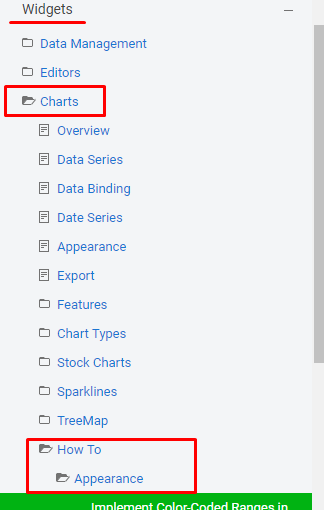# Bar chart customization

1. ##### Fabrizio23 posts
Member since:
Nov 2013

Posted 21 Aug 2018 Link to this post

Hi, I realized the 2 bar chart in a single mvc5/bootstrap page as shown in the image "actual.jpg" (see the code) I would like to obtain the result in "wanted.jpg" in short an overlapping bar chart and changing categories colors, there is a way to do this?

code:
<div class="container-fluid">
<div class="row">
<div class="col-xs-18 col-md-12">
@(Html.Kendo().Chart()
.Name("chart-multi")
.Title("First")
.Legend(legend => legend
.Position(ChartLegendPosition.Top)
)
.Series(series =>
{
series
.Column(new int[] { 20, 40, 45, 30, 50 })
.Color("#cc6e38")
.Spacing(-1)
.Name("on battery");
series
.Column(new int[] { 20, 30, 35, 35, 40 })
.Color("#ef955f")
.Name("on gas");
})
.CategoryAxis(axis => axis
.Categories("Mon", "Tue", "Wed", "Thu", "Fri")
.AxisCrossingValue(0, 10)
)
.ValueAxis(axis => axis
.Numeric()
.Title("miles")
.Min(0).Max(100)
)
.ValueAxis(axis => axis
.Numeric("km")
.Title("km")
.Min(0).Max(161).MajorUnit(32)
)
)
</div>
</div>
</div>

<div class="container-fluid">
<div class="row">
<div class="col-xs-18 col-md-12">
@(Html.Kendo().Chart()
.Name("bar-chart")
.Title("Second")
.Series(series =>
{
series.RangeColumn(new double[][] {
new double[] {0, 121.99},
new double[] {38.47, 121.99},
new double[] {16.17, 38.47},
new double[] {0, 16.17}
}).Labels(labels => labels
.Visible(true)
.From(from => from.Template("#=value.from#"))
.To(to => to.Template("#=value.to#")));
})
.CategoryAxis(axis => axis
.Categories("Total", "Done", "Committed", "Uncommitted")
)
.Tooltip(tooltip => tooltip
.Visible(true)
.Template("#= value.to - value.from #")
)
)
</div>
</div>
</div>

2. ##### Tsvetina2481 posts

Posted 23 Aug 2018 Link to this post

Hello Fabrizio,

I see you already used the Spacing setting to put columns one on top of the others, so you just need to make the top column narrower. This can be achieved with a custom visual. But also, to have a look like the on in the image, you would need to switch the two series order:
`@(Html.Kendo().Chart()`
`    ``.Name(``"chart-multi"``)`
`    ``.Title(``"First"``)`
`    ``.Legend(legend => legend`
`        ``.Position(ChartLegendPosition.Top)`
`    ``)`
`    ``.Series(series =>`
`    ``{`
`        ``series`
`            ``.Column(``new ``int``[] { 20, 30, 35, 35, 40 })`
`            ``.Color(``"#ef955f"``)`
`            ``.Name(``"on gas"``)`
`            ``.Spacing(-1);`
`        ``series`
`            ``.Column(``new ``int``[] { 20, 40, 45, 30, 50 })`
`            ``.Color(``"#cc6e38"``)`
`            ``.Name(``"on battery"``)`
`            ``.Visual(``"customVisual"``);`
`    ``})`
`    ``.CategoryAxis(axis => axis`
`        ``.Categories(``"Mon"``, ``"Tue"``, ``"Wed"``, ``"Thu"``, ``"Fri"``)`
`        ``.AxisCrossingValue(0, 10)`
`    ``)`
`    ``.ValueAxis(axis => axis`
`        ``.Numeric()`
`            ``.Title(``"miles"``)`
`            ``.Min(0).Max(100)`
`    ``)`
`    ``.ValueAxis(axis => axis`
`        ``.Numeric(``"km"``)`
`            ``.Title(``"km"``)`
`            ``.Min(0).Max(161).MajorUnit(32)`
`    ``)`
`)`

`<script>`
`    ``function ``customVisual(e) {`
`        ``var ``origin = e.rect.origin;`
`        ``var ``center = e.rect.center();`
`        ``var ``bottomRight = e.rect.bottomRight();`

`        ``var ``gradient = ``new ``kendo.drawing.LinearGradient({`
`            ``start: [0, 1],`
`            ``end: [1, 1],`
`            ``stops: [{`
`                ``offset: 0,`
`                ``color: ``"#cc6e38"``,`
`                ``opacity: 1`
`            ``}, {`
`                ``offset: 0.25,`
`                ``color: ``"#db9973"``,`
`                ``opacity: 1`
`            ``}, {`
`                ``offset: 1,`
`                ``color: ``"#cc6e38"``,`
`                ``opacity: 1`
`            ``}]`
`        ``});`
`        ``var ``offset = (bottomRight.x - origin.x) / 4;`
`        ``var ``path = ``new ``kendo.drawing.Path({`
`            ``fill: gradient,`
`            ``stroke: {`
`                ``color: ``"#939393"``,`
`                ``width: 0.5`
`            ``}`
`        ``})`
`        ``.moveTo(origin.x + offset, bottomRight.y)`
`        ``.lineTo(bottomRight.x - offset, bottomRight.y)`
`        ``.lineTo(bottomRight.x - offset, origin.y)`
`        ``.lineTo(origin.x + offset, origin.y)`
`        ``.close();`

`        ``return ``path;`
`    ``}`
`</script>`

With regard to coloring the second Chart series, there are a few ways to do this, depending on where the colors come from. If they come from a static array with colors, you could try something like this:
`@(Html.Kendo().Chart()`
`     ``.Name(``"bar-chart"``)`
`     ``.Title(``"Second"``)`
`     ``.Series(series =>`
`     ``{`
`         ``series.RangeColumn(``new ``double``[][] {`
`                            ``new ``double``[] {0, 121.99},`
`                            ``new ``double``[] {38.47, 121.99},`
`                            ``new ``double``[] {16.17, 38.47},`
`                            ``new ``double``[] {0, 16.17}`
`         ``})`
`         ``.ColorHandler(``"getColor"``)`
`         ``.Labels(labels => labels`
`             ``.Visible(``true``)`
`             ``.From(from => from.Template(``"#=value.from#"``))`
`             ``.To(to => to.Template(``"#=value.to#"``)));`
`     ``})`
`     ``.CategoryAxis(axis => axis`
`         ``.Categories(``"Total"``, ``"Done"``, ``"Committed"``, ``"Uncommitted"``)`
`     ``)`
`     ``.Tooltip(tooltip => tooltip`
`         ``.Visible(``true``)`
`         ``.Template(``"#= value.to - value.from #"``)`
`     ``)`
`)`

`<script>`
`    ``var ``colors = [``"orange"``, ``"gold"``, ``"limegreen"``, ``"lightskyblue"``];`
`    ``function ``getColor(data) {`
`        ``var ``index = data.index;`
`        ``var ``numColors = colors.length;`

`        ``var ``colorIndex = index % numColors;`
`        ``return ``colors[colorIndex];`
`    ``}`
`</script>`

If you are binding the series to actual data items from a DataSource and the color comes with the data, you can set the ColorField property of the RangeColumn series to the field in the DataSource that contains the color name.

Regards,
Tsvetina
Progress Telerik
Get quickly onboarded and successful with your Telerik and/or Kendo UI products with the Virtual Classroom free technical training, available to all active customers.
3. ##### Fabrizio23 posts
Member since:
Nov 2013

Posted 27 Aug 2018 in reply to Tsvetina Link to this post

Thank You for the info, exactly what I needed.

There is a tutorial or some documentation that focus on custom visual?

Thanks again

4. ##### Alex Hajigeorgieva768 posts

Posted 29 Aug 2018 Link to this post

Hello, Fabrizio,

I am pleased to hear that my colleague Tsvetina was helpful to you.

We have a custom visual example in the official demos at:

Bar Charts
Line Charts

We also have a folder of how to articles which focus on the appearance of the charts and mostly using the drawing API/custom visuals:

https://docs.telerik.com/kendo-ui/controls/charts/how-to/appearance/color-coded-barsOther resources:

Drawing API overview
Drawing API
Drawing functions
Geometry

We can certainly consider adding more resources if you feel that what is available is not enough. Let me know what you think.

Regards,
Alex Hajigeorgieva
Progress Telerik
Get quickly onboarded and successful with your Telerik and/or Kendo UI products with the Virtual Classroom free technical training, available to all active customers.